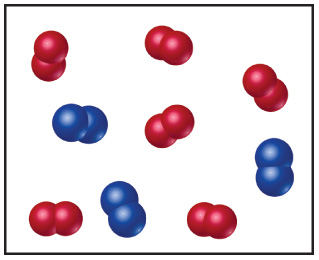# Problem: The following diagram represents the collection of elements formed by a decomposition reaction.If the blue spheres represent N atoms and the red ones represent O atoms, what was the empirical formula of the original compound?

🤓 Based on our data, we think this question is relevant for Professor Seitz's class at UNH.

###### FREE Expert SolutionView Complete Written Solution
###### Problem DetailsThe following diagram represents the collection of elements formed by a decomposition reaction.

If the blue spheres represent N atoms and the red ones represent O atoms, what was the empirical formula of the original compound?